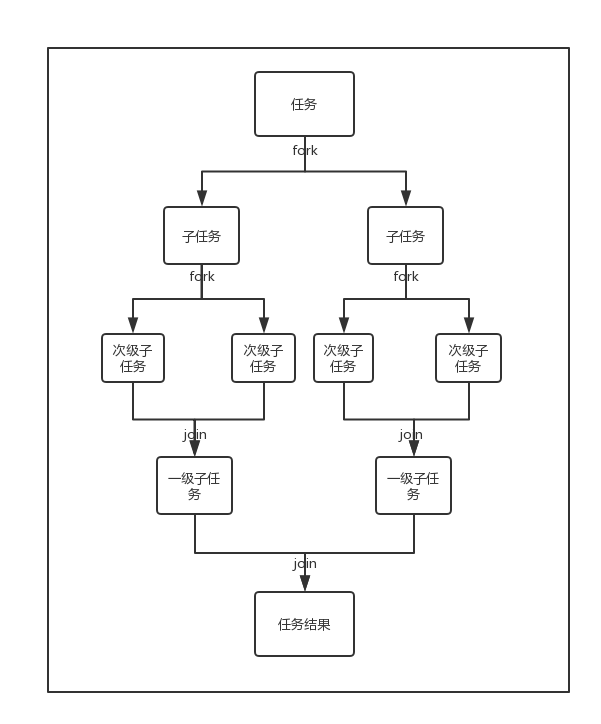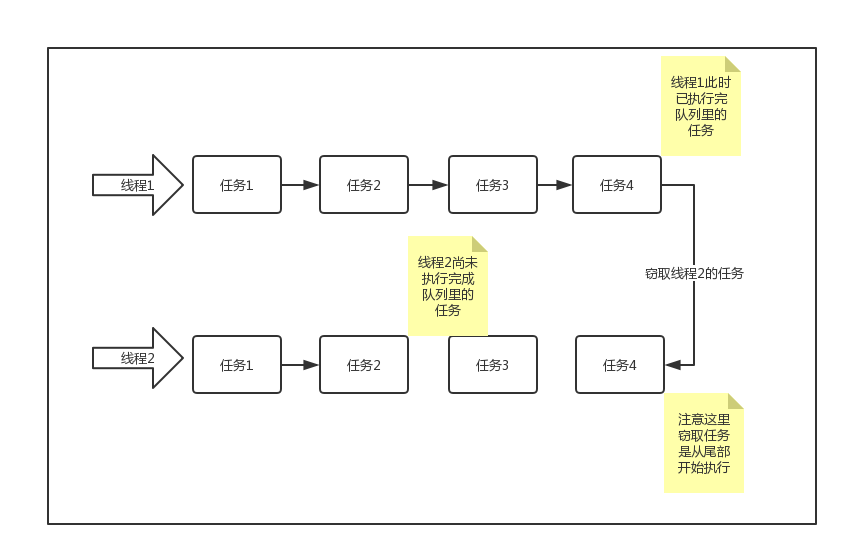# 深入理解Java并发编程之Fork/Join框架

2022年3月23日

## # 什么是Fork/Join

Fork/Join框架是一个实现了ExecutorService接口的多线程处理器，它专为那些可以通过递归分解成更细小的任务而设计，最大化的利用多核处理器来提高应用程序的性能。

fork在英文里有分叉的意思，join在英文里连接、结合的意思。顾名思义，fork就是要使一个大任务分解成若干个小任务，而join就是最后将各个小任务的结果结合起来得到大任务的结果。

Fork/Join的运行流程大致如下所示：``````solve(任务):
if(任务已经划分到足够小):
顺序执行任务
else:
for(划分任务得到子任务)
solve(子任务)
结合所有子任务的结果到上一层循环
return 最终结合的结果
``````

## # 工作窃取算法## # Fork/Join的具体实现

fork()方法:使用线程池中的空闲线程异步提交任务

``````// 本文所有代码都引自Java 8
public final ForkJoinTask<V> fork() {
// 先判断当前线程是否是ForkJoin专有线程，如果是，则将任务push到当前线程所负责的队列里去
else
// 如果不是则将线程加入队列
// 没有显式创建ForkJoinPool的时候走这里，提交任务到默认的common线程池中
ForkJoinPool.common.externalPush(this);
return this;
}
``````

join()方法：等待处理任务的线程处理完毕，获得返回值。

``````public final V join() {
int s;
// doJoin()方法来获取当前任务的执行状态
if ((s = doJoin() & DONE_MASK) != NORMAL)
// 任务异常，抛出异常
reportException(s);
// 任务正常完成，获取返回值
return getRawResult();
}

/**
* doJoin()方法用来返回当前任务的执行状态
**/
private int doJoin() {
int s; Thread t; ForkJoinWorkerThread wt; ForkJoinPool.WorkQueue w;
// 先判断任务是否执行完毕，执行完毕直接返回结果（执行状态）
return (s = status) < 0 ? s :
// 如果是，先判断任务是否处于工作队列顶端（意味着下一个就执行它）
// tryUnpush()方法判断任务是否处于当前工作队列顶端，是返回true
// doExec()方法执行任务
(w = (wt = (ForkJoinWorkerThread)t).workQueue).
// 如果是处于顶端并且任务执行完毕，返回结果
tryUnpush(this) && (s = doExec()) < 0 ? s :
// 如果不在顶端或者在顶端却没未执行完毕，那就调用awitJoin()执行任务
// awaitJoin()：使用自旋使任务执行完成，返回结果
wt.pool.awaitJoin(w, this, 0L) :
externalAwaitDone();
}
``````

### # ForkJoinPool

ForkJoinPool管理着执行池中的线程和任务队列，此外，执行池是否还接受任务，显示线程的运行状态也是在这里处理。

``````@sun.misc.Contended
public class ForkJoinPool extends AbstractExecutorService {
// 任务队列
volatile WorkQueue[] workQueues;

// 线程的运行状态
volatile int runState;

// 公用的线程池，其运行状态不受shutdown()和shutdownNow()的影响
static final ForkJoinPool common;

// 私有构造方法，没有任何安全检查和参数校验，由makeCommonPool直接调用
// 其他构造方法都是源自于此方法
// parallelism: 并行度，
// 默认调用java.lang.Runtime.availableProcessors() 方法返回可用处理器的数量
private ForkJoinPool(int parallelism,
ForkJoinWorkerThreadFactory factory, // 工作线程工厂
UncaughtExceptionHandler handler, // 拒绝任务的handler
int mode, // 同步模式
String workerNamePrefix) { // 线程名prefix
this.workerNamePrefix = workerNamePrefix;
this.factory = factory;
this.ueh = handler;
this.config = (parallelism & SMASK) | mode;
long np = (long)(-parallelism); // offset ctl counts
this.ctl = ((np << AC_SHIFT) & AC_MASK) | ((np << TC_SHIFT) & TC_MASK);
}

}
``````

#### # WorkQueue

ForkJoinPool与传统线程池最显著的区别就是它维护了一个工作队列数组（volatile WorkQueue[] workQueues，ForkJoinPool中的每个工作线程都维护着一个工作队列）。

#### # runState

ForkJoinPool的运行状态。SHUTDOWN状态用负数表示，其他用2的幂次表示。

## # Fork/Join的使用

1, 1, 2, 3, 5, 8, 13, 21, 34, 55, 89······

``````public class FibonacciTest {

class Fibonacci extends RecursiveTask<Integer> {

int n;

public Fibonacci(int n) {
this.n = n;
}

// 主要的实现逻辑都在compute()里
@Override
protected Integer compute() {
// 这里先假设 n >= 0
if (n <= 1) {
return n;
} else {
// f(n-1)
Fibonacci f1 = new Fibonacci(n - 1);
f1.fork();
// f(n-2)
Fibonacci f2 = new Fibonacci(n - 2);
f2.fork();
// f(n) = f(n-1) + f(n-2)
return f1.join() + f2.join();
}
}
}

@Test
public void testFib() throws ExecutionException, InterruptedException {
ForkJoinPool forkJoinPool = new ForkJoinPool();
System.out.println("CPU核数：" + Runtime.getRuntime().availableProcessors());
long start = System.currentTimeMillis();
Fibonacci fibonacci = new Fibonacci(40);
Future<Integer> future = forkJoinPool.submit(fibonacci);
System.out.println(future.get());
long end = System.currentTimeMillis();
System.out.println(String.format("耗时：%d millis", end - start));
}

}
``````

``````CPU核数：4

``````

``````// 普通递归，复杂度为O(2^n)
public int plainRecursion(int n) {
if (n == 1 || n == 2) {
return 1;
} else {
return plainRecursion(n -1) + plainRecursion(n - 2);
}
}

@Test
public void testPlain() {
long start = System.currentTimeMillis();
int result = plainRecursion(40);
long end = System.currentTimeMillis();
System.out.println("计算结果:" + result);
System.out.println(String.format("耗时：%d millis",  end -start));
}
``````

``````计算结果:102334155

``````

``````// 通过循环来计算，复杂度为O(n)
private int computeFibonacci(int n) {
// 假设n >= 0
if (n <= 1) {
return n;
} else {
int first = 1;
int second = 1;
int third = 0;
for (int i = 3; i <= n; i ++) {
// 第三个数是前两个数之和
third = first + second;
// 前两个数右移
first = second;
second = third;
}
return third;
}
}

@Test
public void testComputeFibonacci() {
long start = System.currentTimeMillis();
int result = computeFibonacci(40);
long end = System.currentTimeMillis();
System.out.println("计算结果:" + result);
System.out.println(String.format("耗时：%d millis",  end -start));
}
``````

``````计算结果:102334155

``````